# Character Recognition using Fuzzy Hamming Distance

A project by Marc Lanctot and Hani Ezzadeen presented to
Godfried Toussaint for COMP 644: Pattern Recognition, Winter 2004.

## Introduction

This project is based on the Fuzzy Hamming Distance concept which was taken from this paper .

The Hamming distance (HD) is a dissimilarity measure between bit strings of equal length. Given two bit strings, b1 and b2, the Hamming distance can be defined as the number of differences between bit values of corresponding bit positions in each bit string. Equivalently, it is the number of 1s in b1 XOR b2.For example, let b1 = 11010 and b2 = 01011. In this case, HD(b1,b2) = 2.

Although the Hamming distance has several applications, the main concern is the assumption that every bit is independent of its neighbours. In other words, it assumes no local correlation. In this paper, a new metric is introduced to better deal with bit string patterns which may be locally-correlated. It is referred to as the Fuzzy Hamming distance.

In this project, we propose two distant metrics based on the Fuzzy Hamming Distance introduced in the paper. We apply the metrics in the extended domain of Character Recognition where characters are represented as 2-dimensional bit maps. By measuring the distance between an input character and each character in the "ideal" set and then choosing the "optimal" match, i.e. the character which is most similar to the input character, we provide a tool for character recognition.

An interactive Java applet originally written by  is used as a board for reading the input characters.

## Fuzzy Hamming Distance metrics for Character Recognition

The Fuzzy Hamming Distance (FHD) also measures dissimilarity between bit strings of equal length. However, it is not simply the number of 1s in the result of an XOR operation. The FHD incorporates bit locality: 2 bit strings with a bit displaced by 1 position should not be as distant as 2 incorrect bits. For example, let b1 = 100000011 and b2 = 100000101. Then HD(b1, b2) = 2, even though the only difference is that there is a 1-bit that is off by one position. Now assume that each bit in the bit string represents a quarter-note of time in a muscial pattern, where 1s are tap beats and 0s are silent beats. In this case, b1 and b2 are almost the same. A more intuitive measure for a distant between b1 and b2 would then imply that dist(b1, b2) < 2.

The authors define 3 operations on bit strings that will be needed to define FHD.
• insertion: 'flip' a bit from 0 to 1
• deletion: 'flip' a bit from 1 to 0
• shift: 'move' a 1 bit from position i to position j
Each operation has a constant cost. The constants used by the authors suggest that the cost for an insertion and deletion, ci and cd respectively, are both equal to 1, and that the cost for shift, cs, should be proportional to the difference in bit positions, i and j. That is, ci = cd = 1, and that cs = abs(i-j)*A, where A is arbitrarily chosen so that at some threshold point it becomes cheaper to insert and delete (the authors suggest A = 0.15 is a good choice, in practice). The FHD is defined as the optimal cost to change b1 into b2 using only these operations. Since the optimal cost can be written as a minimum of the cost of a current operation plus the cost of getting to a giving configuration, dynamic programming can be used to build a table of optimal costs up to the configuration where both bitstrings are equal. In the worst case, this takes O(N,M) time, where N is the number of 1-bits in b1 and M the number of 1-bits in b2.

Below is an introductory applet that will demonstrate how the Fuzzy Hamming distance between 2 strings is obtained via the optimal operations. Please input two bitstrings of equal length:

Please get a Java compatible browser to see this.

The FHD is a 1-dimensional metric. For character recognition, we must extend these dissimilarity measures to 2-dimensional. Below, we propose 2 such means of doing so. We assume that you can only measure the distance between 2 bitmaps if they have the same number of rows and columns.

### FlatCrossFHD: A composite metric

FlatCrossFHD (FCFHD) simply flattens the 2D bitmap by considering only its rows and columns. Therefore, given 2 bitmaps, the FCFHD compares all corresponding rows and columns in both bitmaps, each of which are bit strings, using the regular FHD. The FCFHD is the sum of all individual FHDs computed.

### 2DFHD: An extended metric

The 2DFHD metric considers each bitmap as a finite plane of points which can take the values in {0,1}. The operations and costs are as before except that the shift operation is now allowed to move bits up and down instead of only right and left. The cost of a shift operation is precisely the Manhatten distance between positions: the minimum number of "hops" that must be taken from position (i1,j1) to position (i2,j2), where diagonal hops disallowed. As before, the 2DFHD is the optimal cost to change one bitmap to the other using only these operations. It is straight-forward to extend the dynamic programming algorithm presented in the paper to include shifting bits up and down.

## Procedural/Algorithmic Stages

To evaluate the value of these metrice, in practice, we have implemented a Java applet that recognizes characters based on these metrics. The algorithms used in the applet and the basic flow of how our application is modelled is described below.

### (1) Building the Ideal Characters Bitmaps

Each character in the alphabet will be represented as a 100x100 bitmap stored in a PBM file format. The characters images were generated by The Gimp , a common graphics imaging tool, using a 72 pt. Verdana Sans Serif font. Before any comparisons are made with the input characters, each ideal character map is first loaded from the file into an internal data structure. These bitmaps are then skeletonized using Hilditch's algorithm .

### (2) Preprocessing Stage of the Input Characters

The purpose of this stage is to "normalize" the user input, i.e scale it to a predefined size of 100x100 bitmap. This step is crucial to be able to calculate the Fuzzy Hamming distance of the input character relative to bitmaps of the “ideal” characters.

The scaling stage will be addressed shortly, but before we start this stage we need to deal with an intrinsic property to the applet design: the low number of samples of the user input.

#### Increasing the number of Samples of the 2D bitmaps

When the user draws a character, the applet records a finite set of points while the mouse is being dragged then connects these points by straight lines giving the illusion of sampling infinitely many points. The number of recorded points depends on the speed at which the characters are drawn. That is, if the user is drawing at a fast pace, the number of points recorded will be less than the number of points in the case of a slow pace. To handle this problem, all the points necessary to form an 8-connected straight line between any two consecutively recorded points are calculated using linear interpolation. For demonstration purposes, the points recorded by the applet will turn to red once the recognize button in the applet is pressed (see figure (1) ).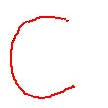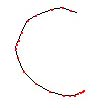Figure (1): The left 'C' character was drawn slowly;
whereas the right one was drawn fast

#### Scaling 2D Bitmaps

We need to scale all user input, regardless of its size, to 100x100 bitmap (see figure (2.b) ). Trying to avoid this stage by simply limiting the size of the applet window to this size will not solve the problem of normalizing the input characters because the user can still draw a miniature character. In any case, scaling is a necessary step.

Scaling has been implemented using two interpolation methods inspired by : nearest neighbor and bi-linear interpolation. Even though nearest neighbor is simpler, its output is not as smooth as the bi-linear interpolation.

Since the pixels of the characters will be 8-connected when scaled, they will remain connected afterwards regardless of the scaling factor. The tradeoff, however, is a variation in the thickness, in terms of the number of pixels, of the resulting characters. This thickness will vary depending on the interpolation and scaling stages. To overcome this variation problem, each character will be skeletonized using Hilditch’s algorithm . See figure (2.c)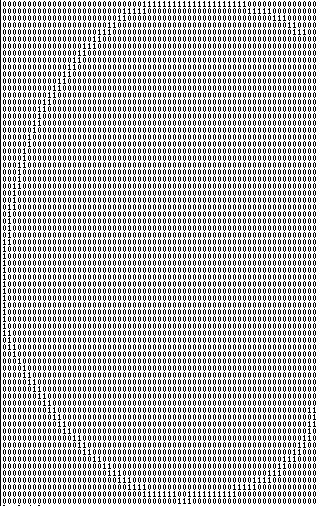(a)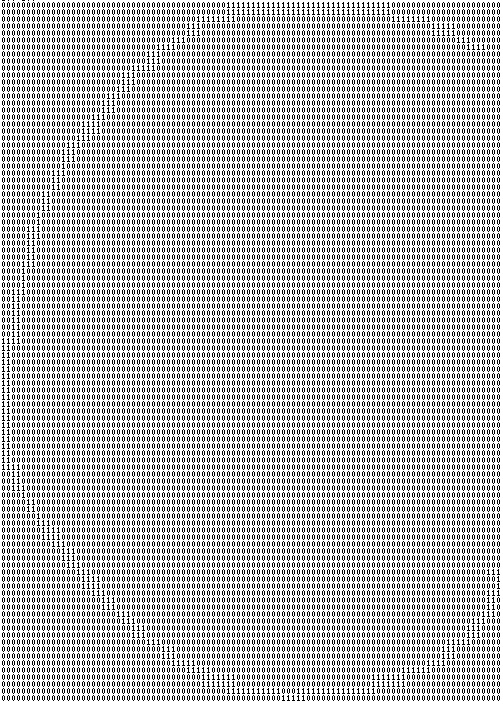(b)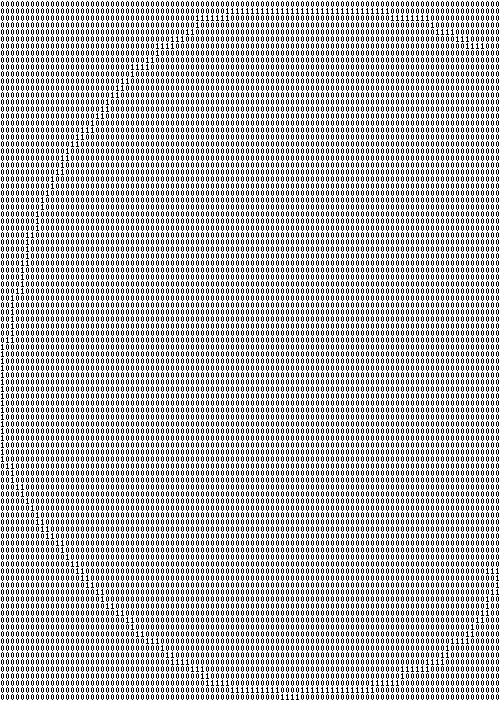(c) Figure (2): a) The input 'C' character. It has a bounding box of size 72 x 63. b) The input 'C' character after scaling to 100 x 100. c) The scaled input 'C' character after applying Hilditch's algorithm. Note that the ones are for the foreground and the zeros are for the background.

### (3) Recognition Stage

In the recognition stage, one of two methods can be implemented. The first method applies the FCFHD metric and the other one applies the 2DFHD metric. In both cases, the procedure for recognizing the character is the same. The distance between the input character's bitmap to each ideal character's bitmap is calculated using the chosen metric.

Finally, the method returns a list of distances, one for each character. The character which gives the smallest Fuzzy Hamming distance relative to the input character is the one that is chosen as the output of the recognition process.

## Implementation

Please draw an UPPERCASE character into the drawing board below.

Please get a Java compatible browser to see this.

### Optimizations

The ImageTools class caches loaded images for quick access. Also, a speed improvement was quickly appreciated when we realized that we could cache the skeletons of the ideal characters: this saves time because we no longer have to pass each image through Hilditch's algotirthm to obtain the skeletons before comparing the letter bitmaps.

### Results/Realizations

One observation is that while these metrics may promise accuracy, they are unfortunately quite computationally expensive. This is more so for 2DFHD than FCFHD. This is impractical and makes character recognition too slow to perform in real-time.

As a result, it is likely that the FHD is more suitable for 1-dimensional problems. The results given by the authors in the paper seem to support this.

## References

 Fuzzy Hamming Distance: A new Dissimilarity Measure.
Abraham Bookstein, Shmuel Tomi Klein, Timo Raita.

 Rune Andre Melgaard, Mads Hansen, Chao-Ching, Mai Burner imageprocessing package.

 Brian Rogers: hw3s rc.txt

 The Gimp, www.gimp.org

 Hilditch's Algorithm. COMP 644 class notes